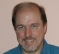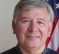## Frequency multiplication in the digital domainStarted by 6 years ago11 replieslatest reply 6 years ago574 views
I need some DSP advice on how to.

I have an input sine waveform that varies in the range from 10Hz to 100kHz.
I need to multiply the frequency of the input sine in discrete steps of either x32, x64, x128 etc.
I can do this in the analog domain with PLL or analog multipliers but it very quickly becomes complex and expensive to do. I'm considering doing this in the digital domain using DSP.
One approach is to double the frequency by multiply the waveform with itself and repeating the process as many times necessary to achieve the desired frequency. The output frequency needs to precise. Not necessarily in phase but without phase drift.

Are there DSP tricks for sine frequency multiplication which I haven't considered?
Additionally, are there tricks for generating a cos from a sin input?

David.

[ - ]if I understood you just generate in an NCO 32,64,128 Hz and multiply by input.

Kaz

[ - ]with more effort to understand your post I think two NCOs may do.

one NCO use to lock in closed loop to input frequency(e.g. 10Hz). second NCO open loop to generate 10*32 HZ once NCO1 is locked i.e. just increment NCO2 accumulator by 10/32 scaled suitably

Kaz

[ - ]If your sampling rate is a good bit above your maximum frequency, and if the noise level is reasonably low, then you can do a first-cut frequency estimation by timing zero crossings or some such, and then lock onto the signal with a PLL.

Given your PLL phase, you could easily synthesize your N-times-frequency sine wave.

The wide range of frequencies will be a challenge -- but not nearly as much as trying to do it in analog.

You do realize that you're implying that you'll be sampling sine waves down to 10Hz, and generating them at 12.8MHz -- that implies a sampling rate well in excess of 25.6MHz (I suggest no less than 50MHz), which means that at the slow end one cycle of your PLL fundamental will take 5 million samples.  It's going to take some careful design to make it work.

[ - ]If you know the frequency, it is easy to delay a sinusoid by a quarter cycle (and negate) to turn sin into cos.  Just make a first-order allpass filter having a quarter-cycle phase delay.

[ - ]For frequency shifting, filter out the negative-frequency component and multiply by a similar "complex sinusoid" tuned to the "difference frequency", thereby summing their frequencies to get the frequency you want, and then take the real part for your final result.

[ - ]Is that all there is in the input?  A pure noiseless sinewave?

[ - ]Since there's a difference between frequency multiplication and frequency shifting, it's likely we need to ask which you want to do.  If there's any modulation of this "sinusoid" it will make a different.

[ - ]Yes it is a pure low noise sine waveform. The input frequency is variable between the said frequencies but I think it might be impractical to cover such a wide band.

The sample rate doesn't have to be fixed. It can slide with the input frequency. I just need enough samples to do the multipliction.

The object is to create sample pulses for another process. I need to do synchronous sampling of the same sine wave. Say 32 samples per cycle. The process does allow for these samples to be acquired over multiple cycles of the input sine wave. For high frequency the process can be under sampled. This requires generating the sample timing for under sampling. For 32 samples per cycle the formula fin - fin/32 works. This produces a frequency lower than the input frequency which coincides in phase every 32 cycles of input. Detection of the zero crossing of the lower frequency will generate sample pulses at 32 discrete phases over 32 cycles of the input sine wave.

The question is how to accurately generate this under sampled sample frequency.

Example, if Fin = 1000Hz then 1000Hz - 1000Hz/32 = 968.75Hz. 968.75Hz is the under sampling frequency for 1000Hz input at a rate of 32 sample per cycle.

For low frequencies I think the frequency multiplication is better if the acquisition time is to be reasonable.

[ - ]If I understand, the input is analog. Can you use analog filters to feed different frequency bands to several digital filters? A PSoC can feed multiple channels to DMA. From the DMA array(s) you can do your analysis.

[ - ]